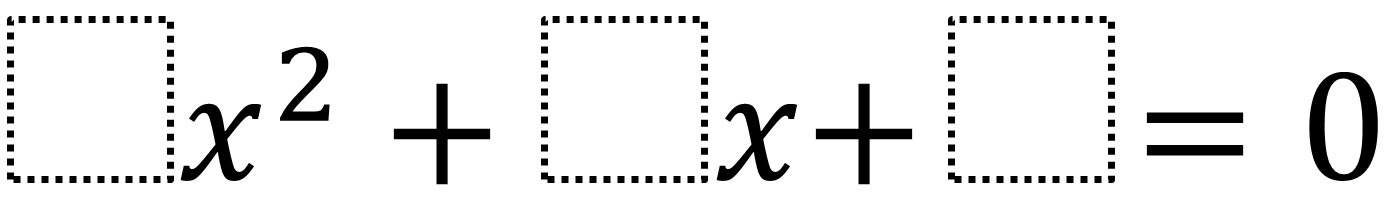Home > High School: Algebra > Factoring Quadratics – One Solution

# Factoring Quadratics – One Solution

Directions: Using the digits 0 to 9 at most one time each, fill in the boxes to so that there is only one solution.### Hint

How could you find the equation if you already knew the solution?

### Answer

There are two answers x^2 + 6x + 9 = 0 which has the solution -3 and 9x^2 + 6x + 1 = 0 which has the solution -1/3.

Source: Daniel Luevanos

## Writing Equivalent Polynomial Expressions

Directions: Use the digits 1-9, at most one time each, to create a true statement. …